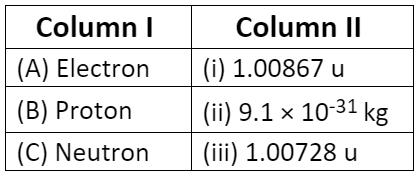Courses

# Test: Structure Of The Atom- 1

## 10 Questions MCQ Test Science Class 9 | Test: Structure Of The Atom- 1

Description
This mock test of Test: Structure Of The Atom- 1 for Class 9 helps you for every Class 9 entrance exam. This contains 10 Multiple Choice Questions for Class 9 Test: Structure Of The Atom- 1 (mcq) to study with solutions a complete question bank. The solved questions answers in this Test: Structure Of The Atom- 1 quiz give you a good mix of easy questions and tough questions. Class 9 students definitely take this Test: Structure Of The Atom- 1 exercise for a better result in the exam. You can find other Test: Structure Of The Atom- 1 extra questions, long questions & short questions for Class 9 on EduRev as well by searching above.
QUESTION: 1

### The charge/mass ratio of the electron______.

Solution:
• British physicist J.J Thomson measured the ratio of electrical charge (e) to the mass (m) of cathode ray particles by using specially designed cathode ray tubes.
• e/m = 1.758820 x 1011 C/Kg
Here m is the mass of the particle of cathode rays in Kg and e is its charge in coulomb C.
• The particles are negatively charged and therefore the charge on the cathode ray particle which was later named as an electron is represented as -e.
• The value of e/m remained constant irrespective of the nature of the gas.
QUESTION: 2

### Dalton’s atomic theory is successfully explained by (i) Law of conservation of mass (ii) Law of constant composition (iii) Law of radioactivity (iv) Law of multiple proportion

Solution:

Dalton's atomic theory proposed that all matter was composed of atoms, indivisible and indestructible building blocks. While all atoms of an element were identical, different elements had atoms of different sizes and mass.

Dalton's atomic theory explains:

1. Law of conservation of mass
2. Law of constant composition
3. Law of multiple proportions
QUESTION: 3

### Rutherford’s scattering experiment fails for very small scattering angles because

Solution:

Rutherford's scattering formula fails for very small scattering angles because due to the strong repulsive force present between the α-particles and the nucleus and also the full nuclear charge of the target atom is partially screened by its electrons.

QUESTION: 4

The conclusion of Rutherford’s scattering experiment does not include

Solution:

From the α scattering experiment, Rutherford concluded that:

1. There is a positively charged centre in the atom called the nucleus. Nearby all the mass of an atom resides in the nucleus.
2. Electrons revolve around the nucleus in well-defined orbitals.
3. The size of the nucleus is very small compared to that of an atom.
(i) Scattering follows Columb's law
(ii) Radius of the nucleus is less than 10−14 m
(iii) α particles can approach with a distance of the order of 10−14 m of the nucleus.
QUESTION: 5

Identify the Mg2+ ion from the following figures where, n and p represent the number of neutrons and protons respectively.

Solution:
• Electronic configuration of 12Mg atom = 2, 8, 2 and that of Mg2+ ion = 2, 8
• Number of protons in Mg atom = 2+ 8 + 2 = 12
• Number of neutrons in Mg atom = 24 -12 = 12
[as mass number of Mg atom = 24 and number of neutrons = mass number – number of protons]

Therefore, option (d) is the correct answer.

QUESTION: 6

When alpha-particles are sent through a thin metal foil, most of them go straight through the foil because_______.

Solution:

When alpha particles are sent through a thin metal foil, most of them go straight through the foil because most part of the atom is empty space. In other words, there is no obstruction in the path of alpha particles.

QUESTION: 7

A student weighs 30 kg. Suppose his body is entirely made up of electrons. How many electrons are there in his body?

Solution:

Weight of 1 electron = 9.1 x 10-31 kg

Let 'x' electrons combine to weigh 30 kg.

∴ (9.1 x 10-31).(x) = 30

Hence, taking the value to R.H.S., we get

x = 3.29 x 1031

QUESTION: 8

Rutherford’s a-particle scattering experiment showed that
(i) electrons have negative charge
(ii) the mass and positive charge of the atom is concentrated in the nucleus
(iii) neutron exists in the nucleus
(iv) most of the space in atom is empty
Which of the above statements are correct?

Solution:

Points (ii) and (iv) are correct. An atom consists of a positively charged, dense and very small nucleus which have all the protons and neutrons. Positive charge is due to protons, as neutrons have no charge. Most of the space is empty because most of the alpha particles pass straight through the gold foil without any deflection.
Electrons have negative charge, it was explained by Thomson. The existance of neutron was discovered by Chadwick.

QUESTION: 9

Which of the following is correct?Solution:
• Rest mass of the electron = 9.1093837015 × 1031 kg
• Mass of a neutron = (1.008665 amu)
• Mass of a proton =  (1.007277 amu)

QUESTION: 10

The ion of an atom has 2 negative charges. Mass number of the atom is 16 and the number of neutrons are 8. What is the number of electrons in the ion?

Solution:

If the charge is negative, add the amount of charge to the atomic number to get the number of electrons. i.e., Here, the atomic mass given is 16, which means atomic no. should be 8
So, 8+2 = 10  is the required answer.